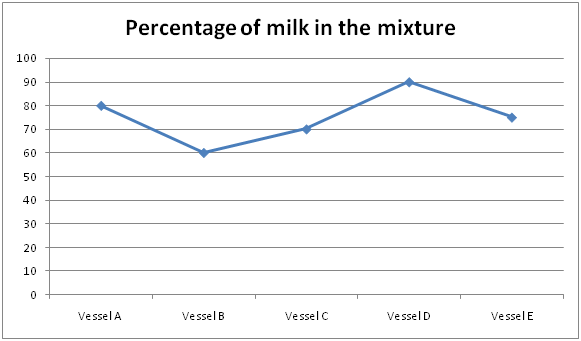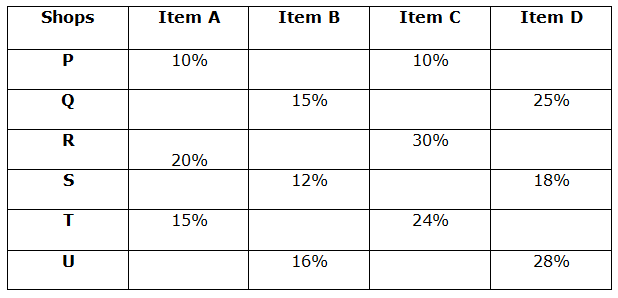# SBI PO Quantitative Aptitude Questions 2019 (Day-18) High Level New Pattern

SBI PO 2019 Notification is about to come and it is the most awaited exam among the aspirants. We all know that new pattern questions are introducing every year in the SBI PO exam. Further, the questions are getting tougher and beyond the level of the candidate’s expectations.

Our IBPS Guide is providing High-Level New Pattern Quantitative Aptitude Questions for SBI PO 2019 so the aspirants can practice it on a daily basis. These questions are framed by our skilled experts after understanding your needs thoroughly. Aspirants can practice these high-level questions daily to familiarize with the exact exam pattern. We wish that your rigorous preparation leads you to a successful target of becoming SBI PO.

[WpProQuiz 5132]

### Click Here for SBI PO Pre 2019 High-Quality Mocks Exactly on SBI Standard

Direction (1 – 5): Study the following information carefully and answer the given questions:

The given line graph shows the mixture of milk in the mixture of water and milk in 5 different vessel.1) If the vessels-A, B and C, each of 400 liter capacity, then what is the ratio of milk and water in the resultant mixture, if all the three vessels mixture is poured into Jar?

a) 7:3

b) 3:5

c) 6:5

d) 5:4

e) None of these

2) There is 1000 liter of mixture in the vessel D. 200 liter of the mixture is taken out and same amount of water is poured into the vessel and again 400 liters of mixture is taken out and same amount of water is poured into vessel, then find the amount of milk in the resultant mixture?

a) 432 liter

b) 456 liter

c) 448 liter

d) 428 liter

e) None of these

3) The Vessel E of 120 liter of mixture of milk and water. 60% of milk and x% of the water is taken out of the vessel. If it is found that the vessels is vacated by 50%, and then find the value of x?

a) 40%

b) 30%

c) 20%

d) 10%

e) None of these

4) A vessel contains mixture of C, x part of the mixture should be withdrawn and replaced by water. If the ratio of the milk and water in the mixture will be 7:18, then find the value of x?

a) 8

b) 7

c) 6

d) Cannot be determined

e) None of these

5) Quantity I: In vessel A, 20 litres of mixture is taken out and 6 litres of water is added in the mixture, then the ratio of milk and water in the final mixture is 8: 3. Find the initial quantity of milk in the vessel

Quantity II: In vessel B, 15 litres of mixture is taken out and 3 litres of water is added in the mixture, then the ratio of water and milk in the final mixture is 7:9. Find the initial quantity of milk in the vessel

a) Quantity I > Quantity II

b) Quantity I < Quantity II

c) Quantity I ≥ Quantity II

d) Quantity I ≤ Quantity II

e) Quantity I = Quantity II (or) Relationship cannot be determined

Directions (6 – 10): Read the following information carefully and answer the given questions. The table below shows the percentage discount allowed by different shops on different items.Note: All the questions are independent from other questions.

6) If the cost price of item B sold by shop Q is Rs. 2040 and the profit percentage earned by the shopkeeper on item B is 20 % more than the discount percentage allowed by the shopkeeper on item B, then find the marked price of Item B sold by shop Q?

a) Rs. 3248

b) Rs. 2456

c) Rs. 2832

d) Rs. 2655

e) None of these

7) If the marked price of item B sold by shop S is twice the marked price of item D sold by the same shop and the total selling price of these items is Rs. 8643 then find the cost price of item B which is sold at a profit of 10%?

a) Rs. 7255

b) Rs. 5875

c) Rs. 4790

d) Rs. 5360

e) Rs. 6475

8) If the marked price of item A and C of shop T is same and the discount allowed on item C is Rs. 192 then find the cost price of item A if the profit percentage earned by the shopkeeper of the shop T in item A is 25 %?

a) Rs. 616

b) Rs. 544

c) Rs. 592

d) Rs. 658

e) None of these

9) If the total discount allowed by the shop P on item C and D is Rs. 1462 and the marked price of item C is Rs. 8500 then find the discount percentage allowed on item D if the selling price of item C and marked price of item D is equal?

a) 14 %

b) 10 %

c) 6 %

d) 12 %

e) 8 %

10) If the cost price of item A sold by shop R is Rs. 16500 and the profit percentage earned by the shopkeeper on selling the item is 2/5 of the discount percentage allowed, then find the marked price of item A?

a) Rs. 22275

b) Rs. 11175

c) Rs. 13397

d) Rs. 12050

e) Rs. 16475

Direction (1-5) :

Milk of vessel A=400*80/100=320 liter

Water of vessel A=400*20/100=80 liter

Milk of vessel B=400*60/100=240

Water of vessel B=400*40/100=160

Milk of vessel C=400*70/100=280

Water of vessel C=400*30/100=120

Required ratio = (320+240+280):(80+160+120)

=840:360

=7:3

Milk=1000*90/100=900 liter

Water=1000*10/100=100 liter

Ratio of the mixture=900:100=9:1

200 liters taken in the mixture,

Milk in remaining mixture = 800*9/10=720

Water in remaining mixture = 800*1/10=80,

Total water=80+200=280

Ratio=720:280=18:7

400 liters is taken out in the mixture, milk=600*18/25=432 liter

Milk=120*75/100=90 liter

Water=120*25/100=30 liter

60% of the milk=90*60/100=54 liter

Remaining milk=90*40/100=36 liter

Remaining water=30*(100-x)/100

Total mixture in the vessel now = (36+30-(3x/10)) = 66-3x/10

(66-3x/10)/120=50/100

(660-3x)/1200=50/100

660-3x=600

3x=60

X=20%

Ratio of mixture of milk to water=7:3 = 7y: 3y

(7y -(7/10*X))/(3y – 3/10x)+x=7/18

The given information is not sufficient to answer the given question. Because we don’t have any information about the initial quantity; instead, we only know the ratio of milk and water in the given mixture.

Quantity I: In vessel A, 20 litres of mixture is taken out and 6 litres of water is added in the mixture, then the ratio of milk and water in the final mixture is 8: 3. Find the initial quantity of milk in the vessel

Milk and water ratio = 80: 20

= 4: 1

Milk in the 20 litres of mixture = 20 * 4/5 = 16 litres

Water in the 20 litres of mixture = 20 * 1/5 = 4 litres

According to the question,

(4x – 16)/(x – 4 + 6) = 8/3

(4x – 16)/(x + 2) = 8/3

12x – 48 = 8x + 16

4x = 16 + 48

4x = 64

X = 16

Initial quantity of Milk in the vessel A = 4* 16 = 64 litres

Quantity II: In vessel B, 15 litres of mixture is taken out and 3 litres of water is added in the mixture, then the ratio of water and milk in the final mixture is 7:9. Find the initial quantity of milk in the vessel

Milk and water ratio = 60: 40

= 3: 2

Milk in the 15 litres of mixture = 15 * 3/5 = 9 litres

Water in the 15 litres of mixture = 15 * 2/5 = 6 litres

According to the question,

(3x – 9)/(2x – 6 + 3) = 9/7

(3x – 9)/(2x – 3) = 9/7

21x – 63 = 18x – 27

3x = 63 – 27

3x = 36

X = 12

Initial quantity of Milk in the vessel A = 3* 12 = 36 litres

Hence, Quantity I > Quantity II

Direction (6-10) :

Profit % = 15*(120/100) = 18 %

According to the question,

CP*[(100+ P%)/100] = [(100- d%)/100]*MP

CP*[(100+18)/100] = [(100 -15)/100]*MP

2040*(118/100) = (85/100)*MP

MP = 2040*(118/100)*(100/85)

MP = Rs. 2832

Let the marked price of item D sold by shop S be x

The marked price of item B sold by shop S = 2x

Selling price of item B= 2x*(100-12)/100 = 2x*88/100

Selling price of item D= x*(100-18)/100 = x*82/100

So,

2x*88/100 + x*82/100 = 8643

258x/100 = 8643

x= 8643*(100/258)

x= 3350

Selling price of item B= 2x*88/100 = (2*3350)*(88/100) = Rs. 5896

Cost price of item B= 5896*100/110= Rs. 5360

Marked price of item A and C of Shop T is same

Marked price of item C of shop T

= 192*(100/24)

= Rs. 800

Marked price of item A

= Rs. 800

Selling price of item A

= 800*(85/100)

= Rs. 680

Cost price of item A

= 680*(100/125)

= Rs. 544

Selling price of item C = Marked price of item D

Discount allowed on item C

= 8500*10/100

= Rs. 850

Selling price of item C= 8500*(90/100) = Rs. 7650

Marked price of item D = Rs. 7650

Discount allowed on item D= 1462 – 850= Rs. 612

MP*(D %/100) = 612

7650*(D %/100) = 612

Discount % for item D = 612*(100/7650) = 8 %

Profit percentage earned on item A sold by shop R

= 2*20/5= 8%

Selling price of item A= 16500*108/100= Rs. 17820

Marked price of item A= 17820*100/80= Rs. 22275

### Click Here for SBI PO Pre 2019 High-Quality Mocks Exactly on SBI Standard